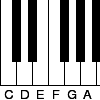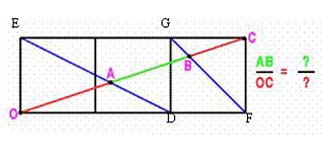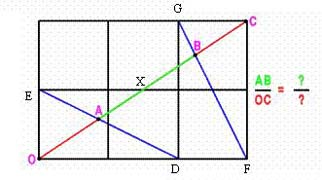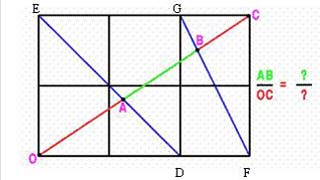You may also likePent

The diagram shows a regular pentagon with sides of unit length. Find all the angles in the diagram. Prove that the quadrilateral shown in red is a rhombus.Tuning and Ratio

Why is the modern piano tuned using an equal tempered scale and what has this got to do with logarithms?Rarity

Show that it is rare for a ratio of ratios to be rational.(1) As triangles AOD and AEC are similar $${OA\over AC} = {OD\over EC} = {2\over 3} {\Rightarrow}{OA\over OC} = {2\over 5}.$$ As triangles BOF and BCG are similar $${OB\over BC} = {OF\over GC} = {3\over 1} {\Rightarrow} {OB\over OC} = {3\over 4}.$$ Hence: $${AB\over OC} = {OB - OA\over OC} = {3\over 4} - {2\over 5} = {7\over 20}.$$(2) From triangles OAD and AXE $${OA\over AX} = {OD\over EX} = {2\over 1.5}= {4\over 3}$$ and hence $${OA\over OX} = {4\over 7} \quad {\rm and} \quad {OA\over OC} = {2\over 7}.$$ Again $${OB\over OC} = {3\over 4}.$$ Hence: $${AB\over OC} = {OB - OA\over OC} = {3\over 4} - {2\over 7} = {13\over 28}.$$(3) The reasoning in this part is identical to part (1).